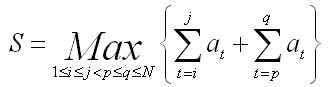poj2593,

## Problem:

Max Sequence
 Time Limit: 3000MS Memory Limit: 65536K Total Submissions: 10971 Accepted: 4507
Description
Give you N integers a1, a2 ... aN (|ai| <=1000, 1 <= i <= N).You should output S.
Input
The input will consist of several test cases. For each test case, one integer N (2 <= N <= 100000) is given in the first line. Second line contains N integers. The input is terminated by a single line with N = 0.
Output
For each test of the input, print a line containing S.
Sample Input
```5
-5 9 -5 11 20
0```
Sample Output
`40`
Source
POJ Monthly--2005.08.28,Li Haoyuan

## Source Code:

//Thu Apr  1 00:33:40 CDT 2010
#include <vector>
#include <list>
#include <map>
#include <set>
#include <deque>
#include <queue>
#include <stack>
#include <bitset>
#include <algorithm>
#include <functional>
#include <numeric>
#include <utility>
#include <sstream>
#include <iostream>
#include <iomanip>
#include <cstdio>
#include <cmath>
#include <cstdlib>
#include <cctype>
#include <string>
#include <cstring>
#include <cstdio>
#include <cmath>
#include <cstdlib>
#include <ctime>

using namespace std;

int main()
{
freopen("input.in", "r", stdin);
freopen("output.out", "w", stdout);
int n, i, max, sum;
int a, b, c;
while (scanf("%d", &n) && n!=0)
{
max = -1000;
sum = 0;
if (n == 1)
{
scanf("%d", &a);
printf("%d\n", a);
continue;
}
if (n == 2)
{
scanf("%d", &a);
scanf("%d", &a);
printf("%d\n", a + a);
continue;
}
//b[i] is the max sum from 0 to i.
for (i = 0; i < n; i++)
{
scanf("%d", &a[i]);
sum += a[i];
if (sum < 0)
sum = 0;
else if (sum > max)
max = sum;
b[i] = max;
}
//c[i] is the max sum from i to the end.
max = -1000;
sum = 0;
for (i = n - 1; i >= 0; i--)
{
sum += a[i];
if (sum < 0)
sum = 0;
else if (sum > max)
max = sum;
c[i] = max;
}
max = 0;
for (i = 0; i < n - 1; i++)
if (b[i] + c[i + 1] > max)
max = b[i] + c[i + 1];
printf("%d\n", max);
}

return (0);
}